低副瓣阵列的设计原理

2022-01-12 来源：微波射频网 作者：94巨蟹座少年 字号：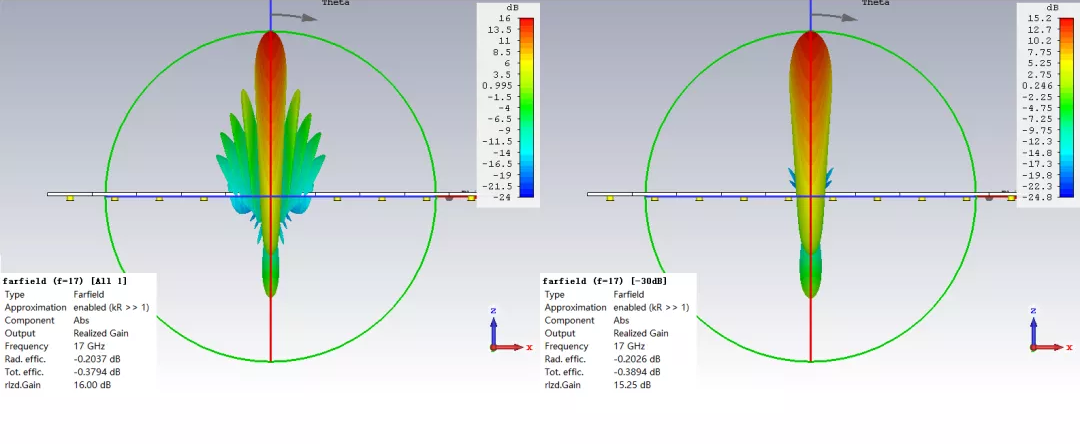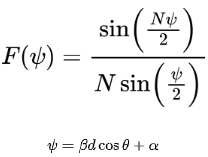%matlab code clear;clc;N=10;format long; x=-pi/2:0.01:pi/2;y=abs(sin(N*x)./(N*sin(x))); %find first sidelobe [value1,id1]=findpeaks(y); [value2,id2]=sort(value1); first_sidelobe=20*log10(value2(end-1)); location=x(id1(floor(length(id1)/2))); plot(x,y,'r','linewidth',2); set(gca,'linewidth',3); xlabel('\psi','Fontsize',12); axis([-pi/2,pi/2,0,1]); h=legend({strcat('$$\left| {{{\sin \left( {N\psi} \right)} \over {N\sin \left( \psi \right)}}} \right|,N=',num2str(N),'$$'),...     },'interpreter','latex'); title({strcat('第一副瓣电平(dB)：',num2str(first_sidelobe));strcat('位置：',num2str(location))}); set(h,'FontName','Times New Roman','FontSize',12,'FontWeight','normal');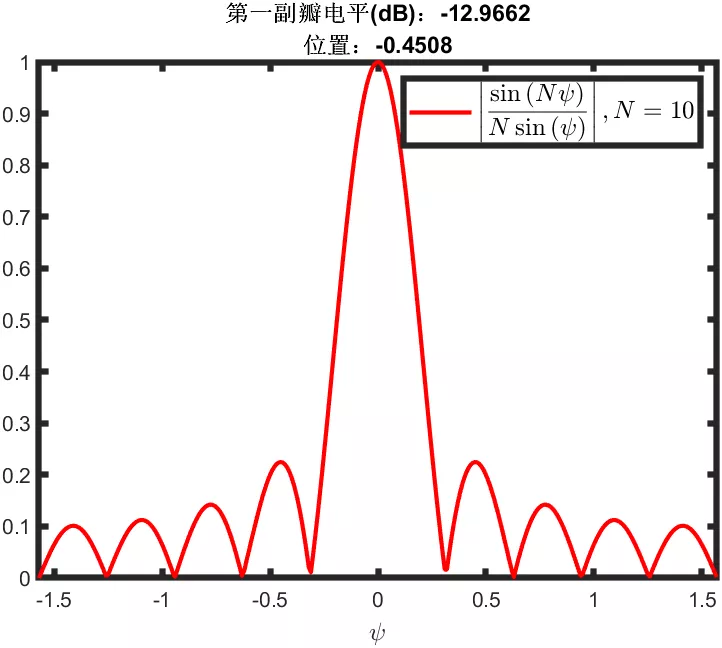其实是单元数N取小了，将上面代码的N赋值为50，将步进间隔由0.01改为0.0001，就会得到下图，基本上达到了理论值。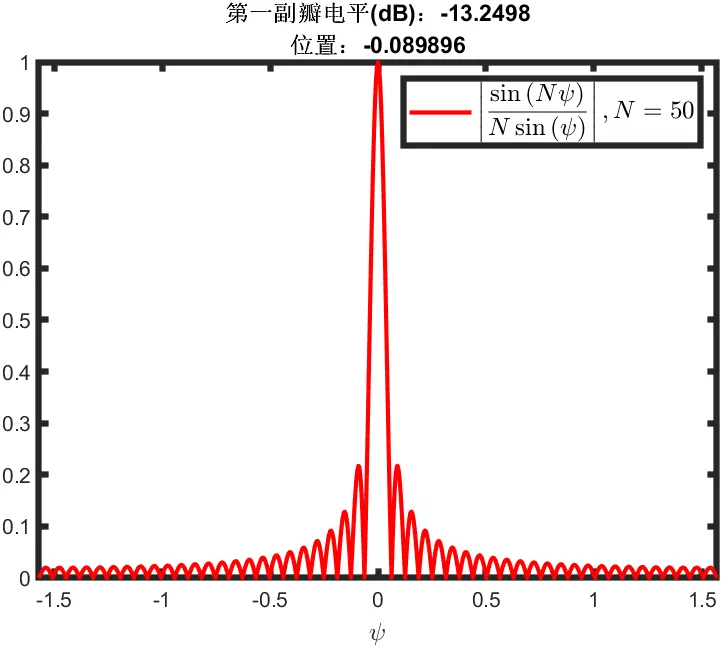虽然与一般教材上的-13.5dB还有0.25dB差值，但这部分是因为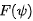这个函数求第一极值点采用了近似计算的方法，第一副瓣位置对应的位置值为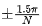与实际副瓣位置点有一定的偏差造成的。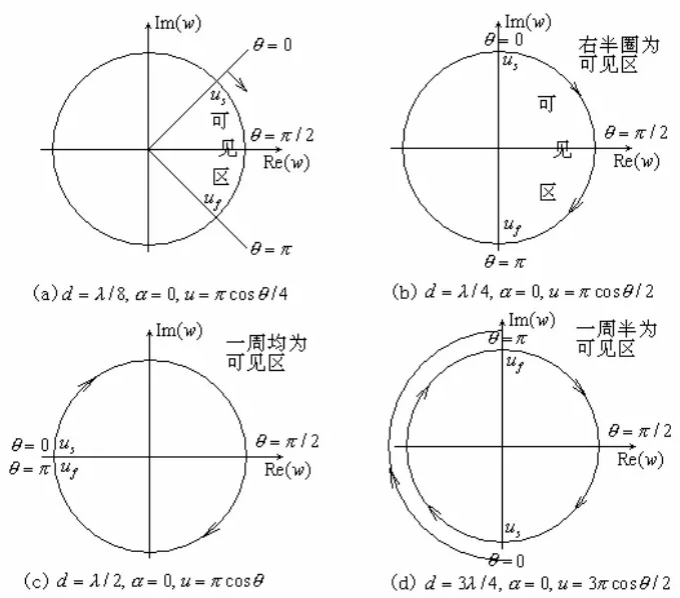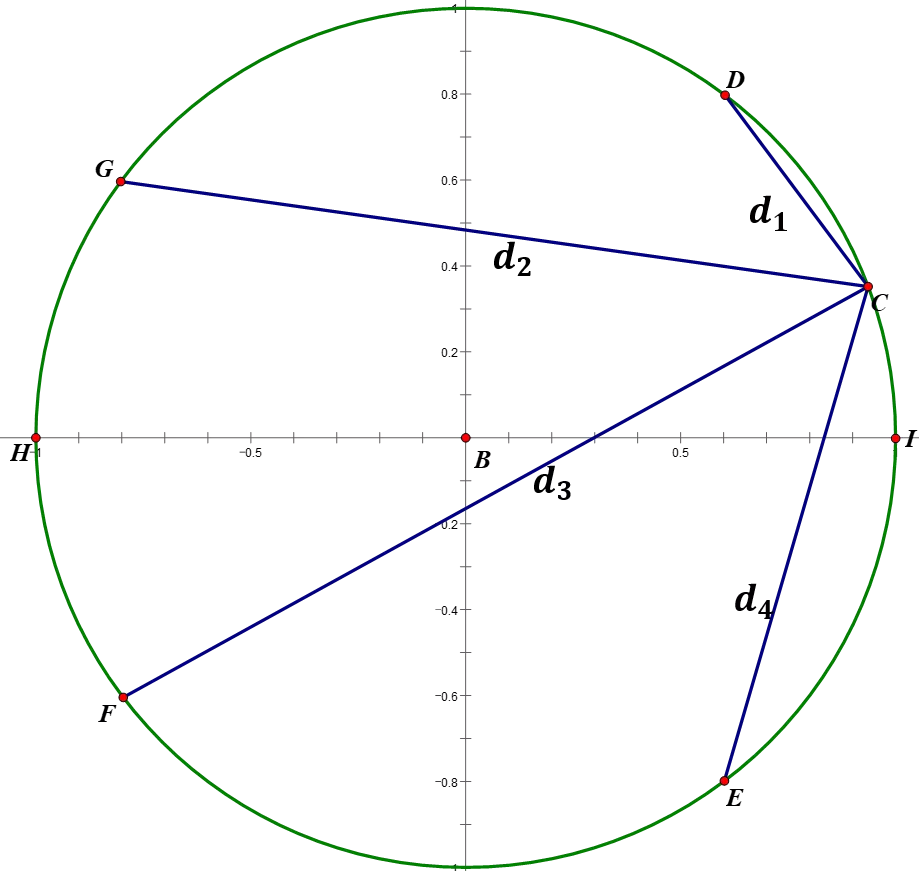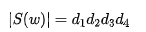(1)  等副瓣电平；

(2)  在相同副瓣电平和相同阵列长度下主瓣最窄，称为最佳阵列；

(3)  单元数多，且副瓣电平要求不是很低时，阵列两端单元激励幅度跳变大，使馈电困难。

·切比雪夫多项式在逼近理论中有重要的应用。这是因为第一类切比雪夫多项式的根（被称为切比雪夫节点）可以用于多项式插值。相应的插值多项式能最大限度地降低龙格现象，并且提供多项式在连续函数的最佳一致逼近。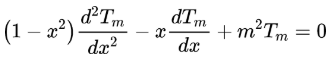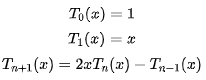x=-1.5:0.01:1.5; T1_x=x; T2_x=2*x.^2-1; T3_x=4*x.^3-3*x; T4_x=8*x.^4-8*x.^2+1; h1=plot(x,T1_x,'r','linewidth',3); hold on; h2=plot(x,T2_x,'g','linewidth',3); h3=plot(x,T3_x,'b','linewidth',3); h4=plot(x,T4_x,'c','linewidth',3); axis([-1.5 1.5 -6 6]); set(gca,'linewidth',3); xlabel('x','fontName','Times New Roman','fontsize',18); ylabel('T_n(x)','fontName','Times New Roman','fontsize',18); %绘制虚线 xx=-1:0.1:1; y1=-1*ones(1,length(xx));y2=1*ones(1,length(xx)); h5=plot(xx,y1,'--k','linewidth',1); h6=plot(xx,y2,'--k','linewidth',1); yy=-1:0.1:1; x1=-1*ones(1,length(yy));x2=1*ones(1,length(yy)); h7=plot(x1,yy,'--k','linewidth',1); h8=plot(x2,yy,'--k','linewidth',1); %图例 legend([h1,h2,h3,h4],'T_1(x)','T_2(x)','T_3(x)','T_4(x)','Location','Best'); hold off;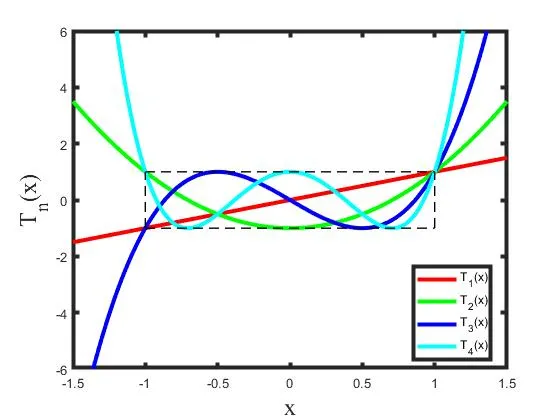• 对于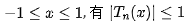。在这个范围内，切比雪夫多项式的函数值在  ±1之间呈现等波纹振荡。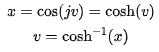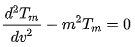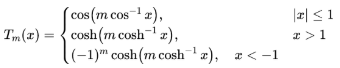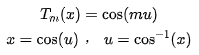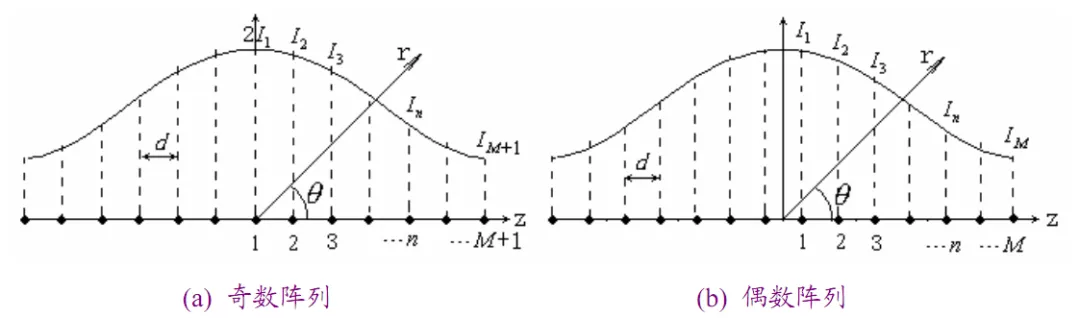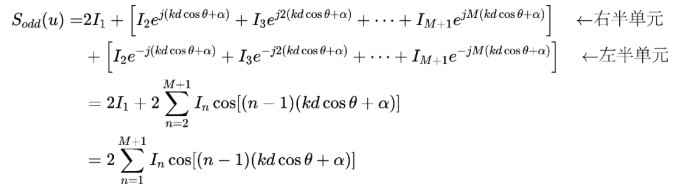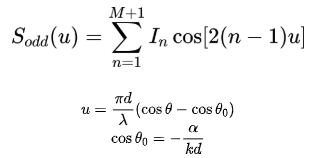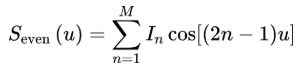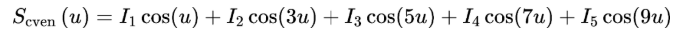无量纲形式的主副瓣比为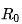采用用变量代换令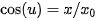其中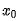满足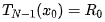这样将cos(nu)用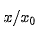进行替换并展开 :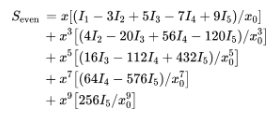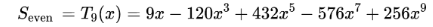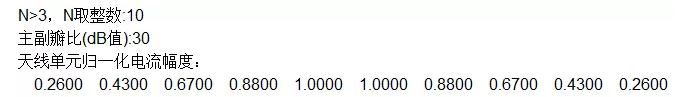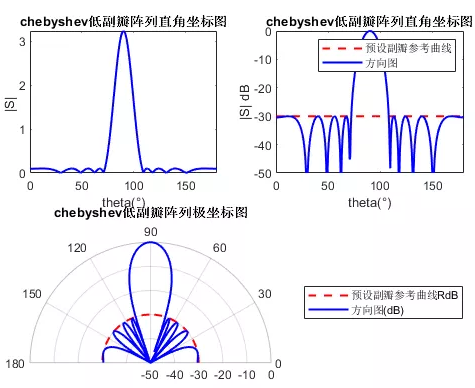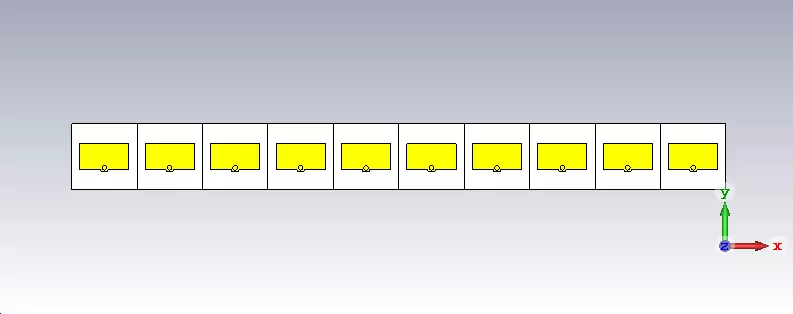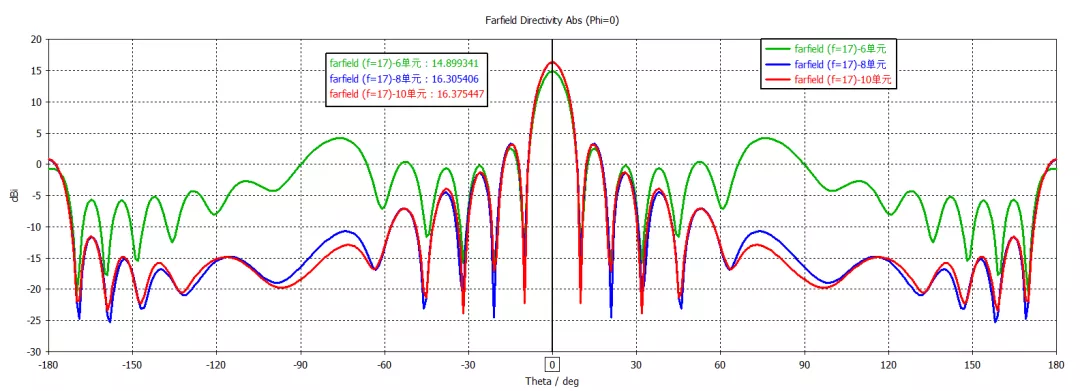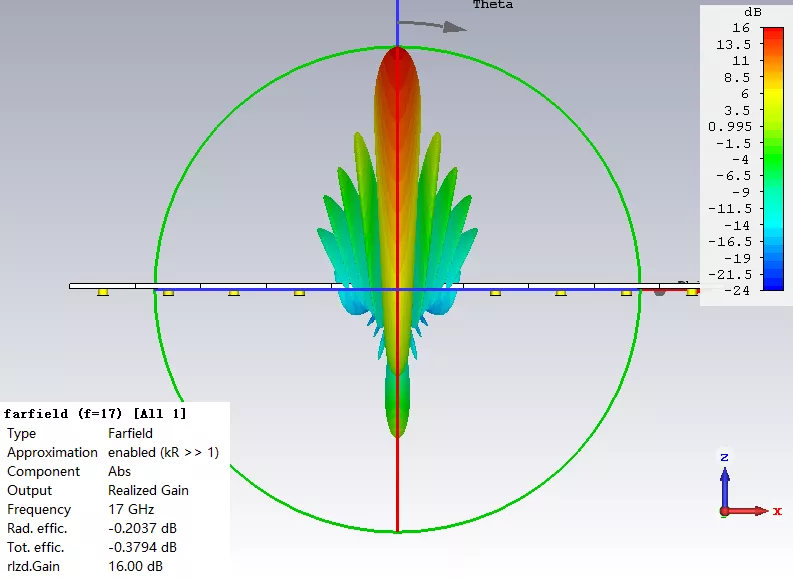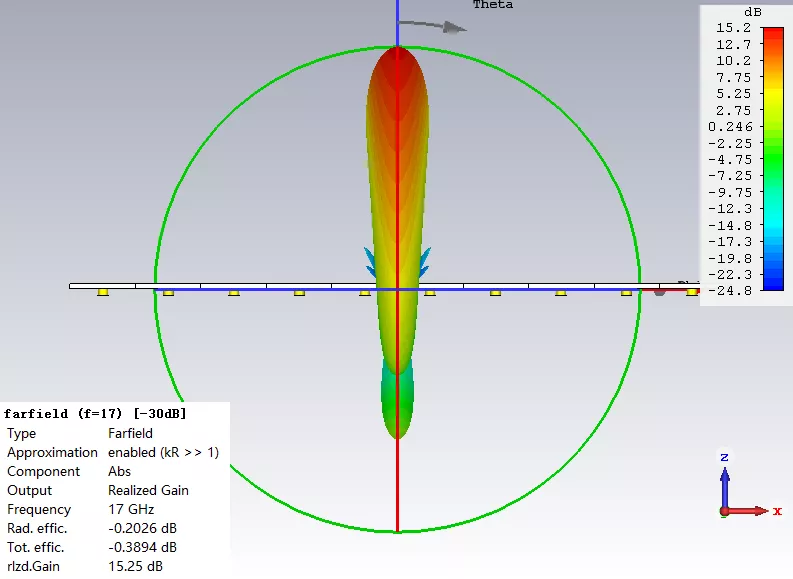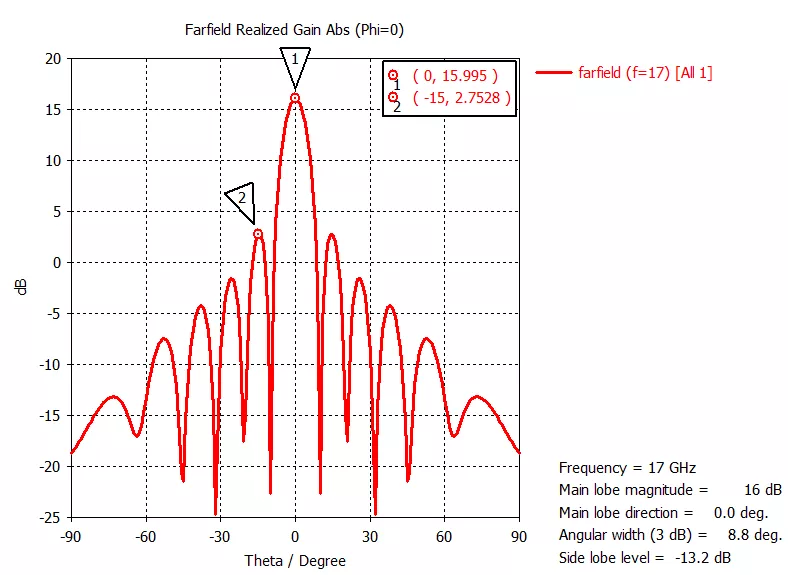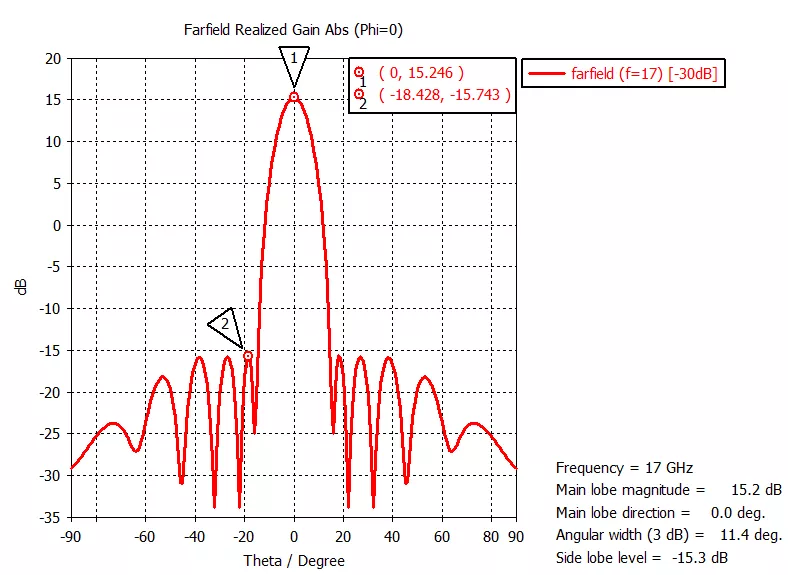PDF文件在下面的总文件夹进行更新：低副瓣阵列的设计原理

https://pan.baidu.com/s/1wi7BcSFhpvdU6lvb2vFqcg

END